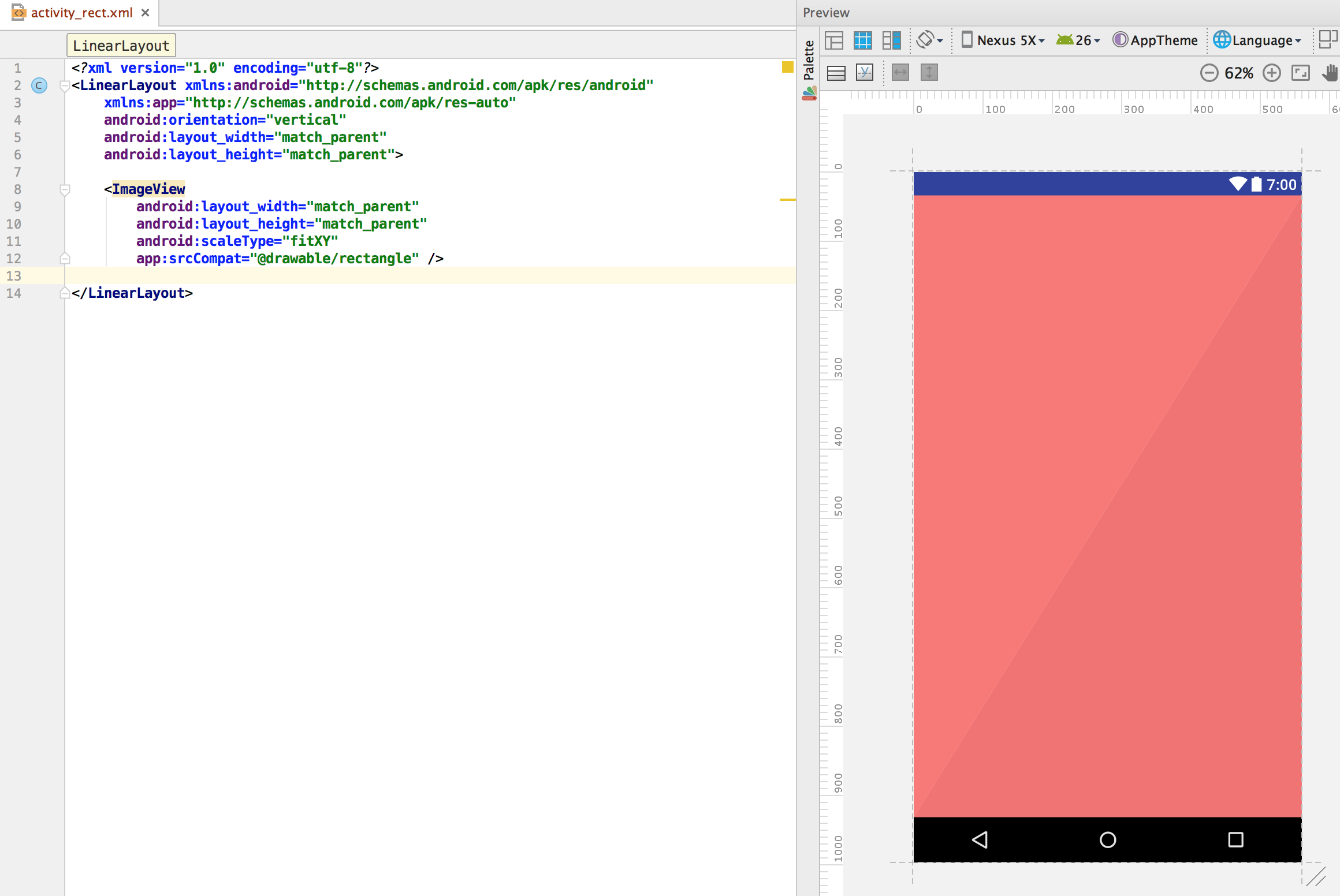# Creating simple vector drawables in Android Studio

Using vector drawables is often much better than bitmap images for two reasons: they scale without losing definition and you need only one asset file which fits all screen densities.

Sometimes I find it useful to create vector drawables by hand in Android Studio using SVG paths.

If you are not yet familiar with it, let me show you an example.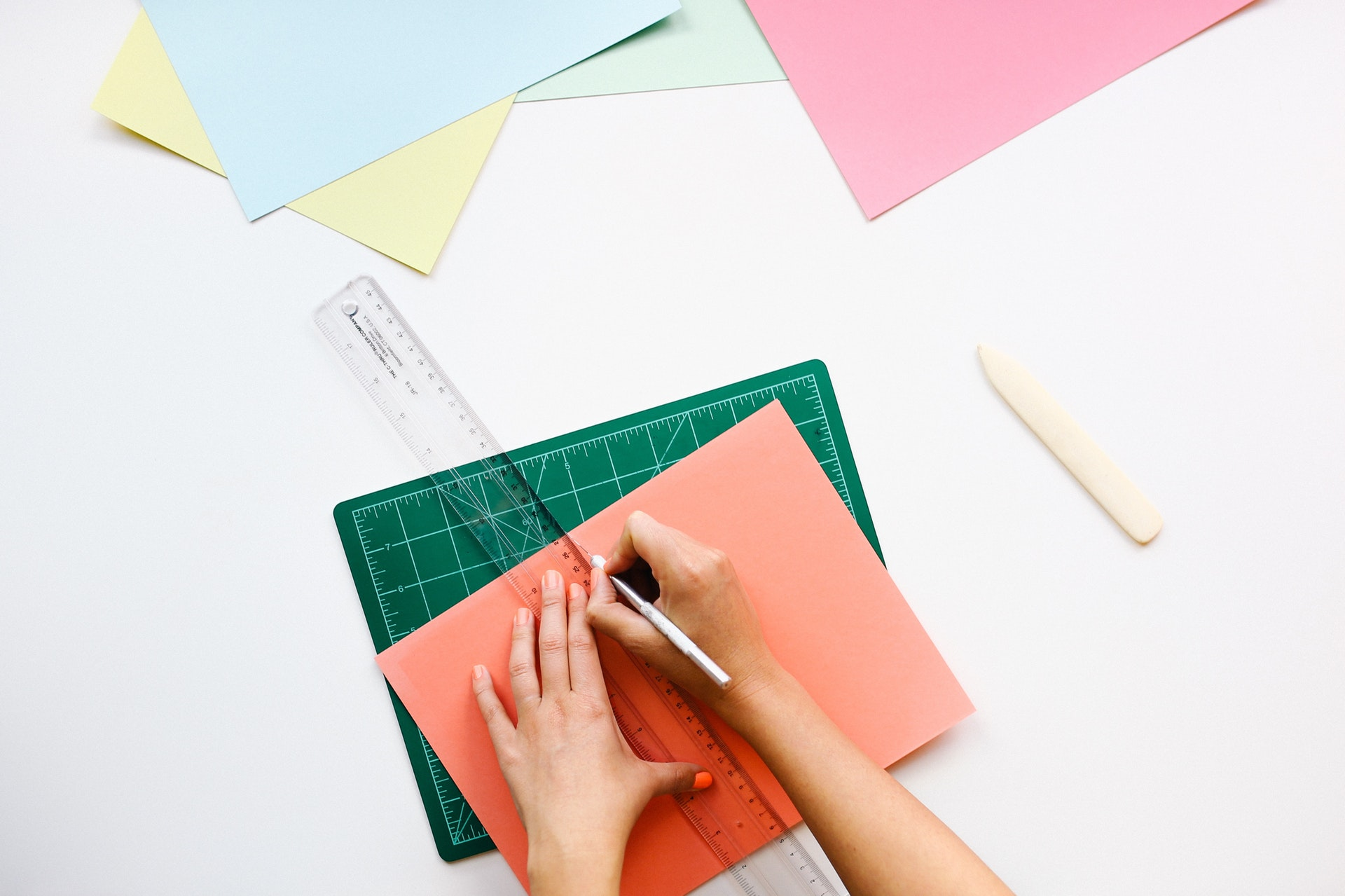# Example

I’m going to create a rectangle consisting of two adjacent triangles varying in color like below: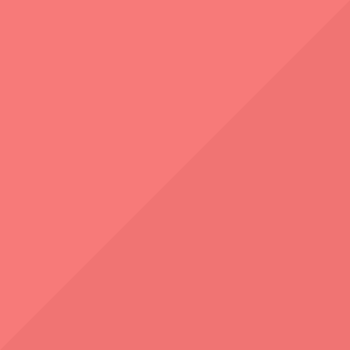## Step 1: Create a drawable file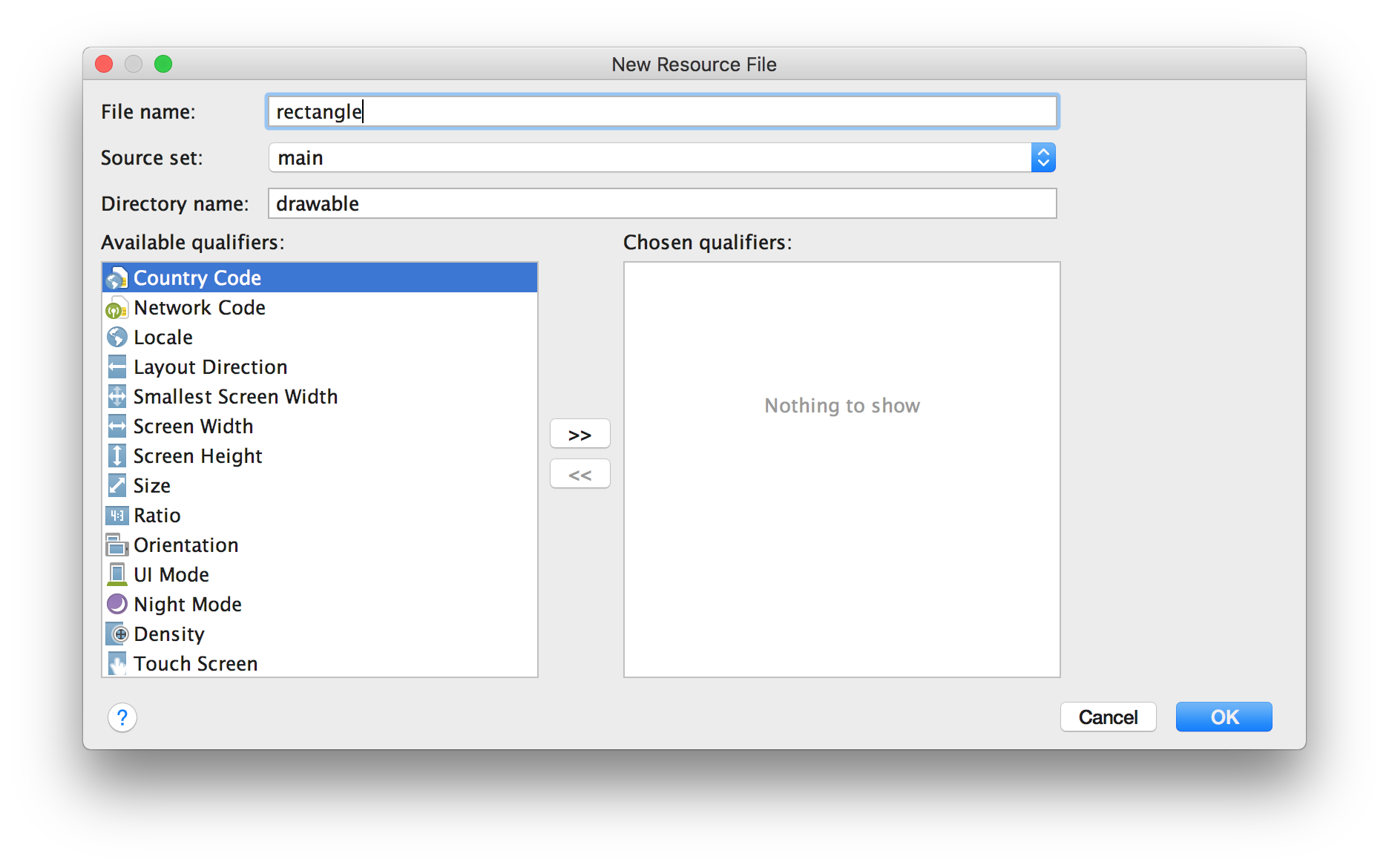## Step 2: Set up the drawable size

``````<?xml version="1.0" encoding="utf-8"?>
<vector xmlns:android="http://schemas.android.com/apk/res/android"
android:height="100dp"
android:width="100dp"
android:viewportHeight="100"
android:viewportWidth="100">

</vector>
``````

The `android:width` and `android:height` properties define the drawable intrinsic size, so it matters mostly when you later use the drawable with `wrap_content` width and height like this:

``````<ImageView
android:src="@drawable/rectangle"
android:layout_width="wrap_content"
android:layout_height="wrap_content" />
``````

The `android:viewportWidth` and `android:viewportHeight` properties are more important for us as they define the canvas size for drawing our paths.

## Step 3: Draw the first triangle

Let’s fill a half of our squared canvas with the first triangle by drawing a path though its vertices: A, B and C.

Please note the coordinates — the top-left corner of the canvas is (0,0) and the bottom-right corner is (100,100) and that’s because we set the `android:viewportWidth="100"` and `android:viewportHeight="100"`.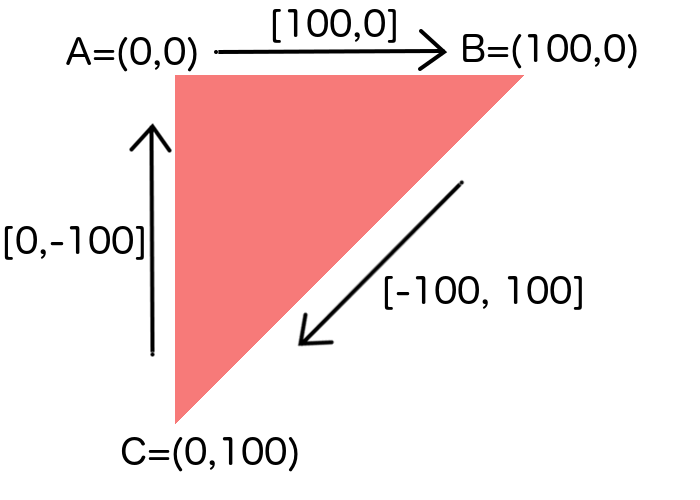You can draw a path by adding a `path` inside the `vector`:

``````<path
android:fillColor="[your color (e.g. from resources or RGB Hex)]"
android:pathData="[SVG path commands]" />
``````

We need the following SVG commands here:

• M — absolute moveto
• L — absolute lineto
• zclosepath

Drawing the triangle consists of:

1. starting a path from A=(0,0): `M 0,0`
2. drawing a line to B=(100,0): `L 100,0`
3. drawing a line to C=(0,100): `L 0,100`
4. closing the path: `z`

We could also use the relative lineto (`l`) command instead and provide the vectors connecting the points like this:

• from A to B: `l 100,0`
• from B to C: `l -100,100`

It’s also worth noting that if you use the same command multiple times in a row, the command letter can be eliminated on subsequent commands. So the final command looks like this: `M 0,0 L 100,0 0,100 z`

Let’s put this in the drawable file:

``````<?xml version="1.0" encoding="utf-8"?>
<vector xmlns:android="http://schemas.android.com/apk/res/android"
android:height="100dp"
android:width="100dp"
android:viewportHeight="100"
android:viewportWidth="100">

<path
android:fillColor="@color/triangle_red_light"
android:pathData="M 0,0 L 100,0 0,100 z" />

</vector>
``````

We can see the triangle in the preview panel: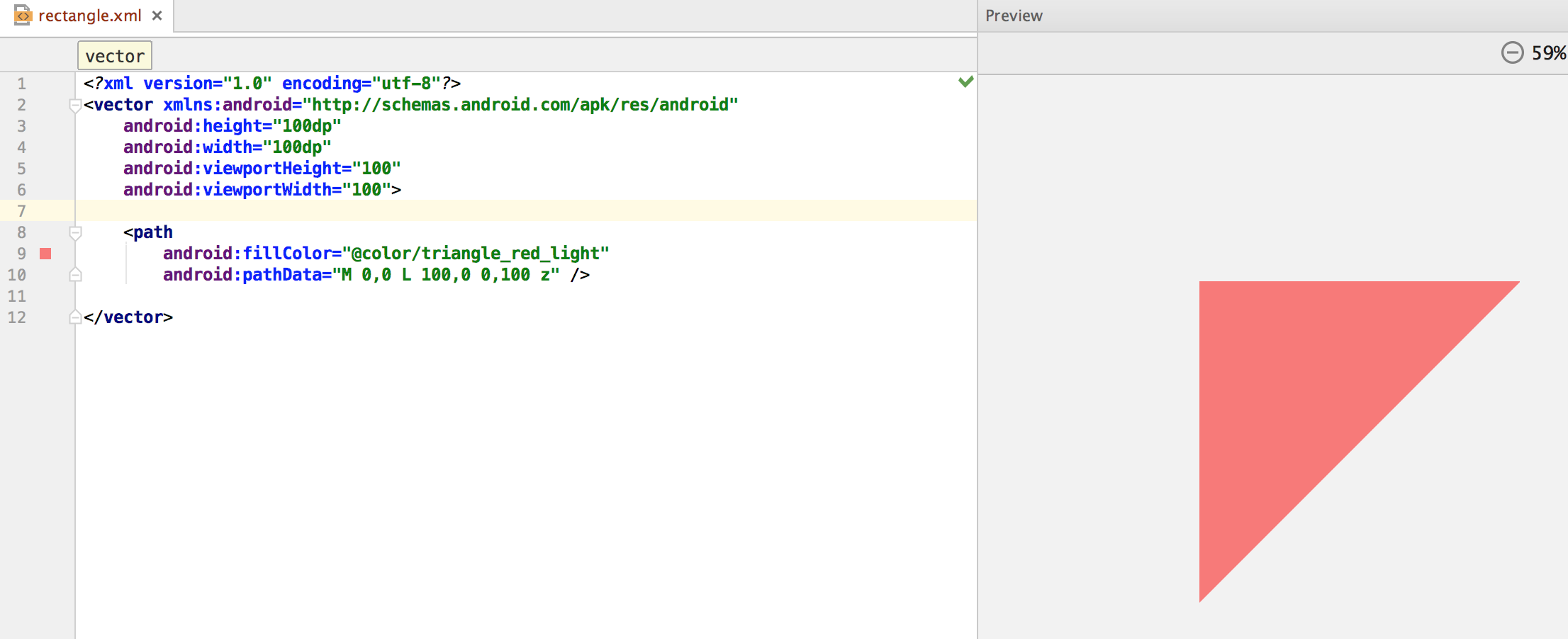## Step 4: Draw the second triangle

As we already know how to draw a path, it’s very simple to create another triangle. Its vertices are:

• B=(100,0)
• C=(0,100)
• D=(100,100)

so the path command is: `M 100,0 L 0,100 100,100 z`

The resulting XML now looks like this:

``````<?xml version="1.0" encoding="utf-8"?>
<vector xmlns:android="http://schemas.android.com/apk/res/android"
android:height="100dp"
android:width="100dp"
android:viewportHeight="100"
android:viewportWidth="100">

<path
android:name="dark_triangle"
android:fillColor="@color/triangle_red_dark"
android:pathData="M 100,0 L 0,100 100,100 z" />

<path
android:name="light_triangle"
android:fillColor="@color/triangle_red_light"
android:pathData="M 0,0 L 100,0 0,100 z" />

</vector>
``````

Hint: you can name the paths so it’s easier to find them later if your drawable gets more complicated.## Step 5: Use the drawable with an ImageView

Now we can use the drawable. As you can see, it can be easily scaled by using the `android:layout_width`, `android:layout_height` and `android:scaleType` properties.

``````<?xml version="1.0" encoding="utf-8"?>
<LinearLayout xmlns:android="http://schemas.android.com/apk/res/android"
xmlns:app="http://schemas.android.com/apk/res-auto"
android:orientation="vertical"
android:layout_width="match_parent"
android:layout_height="match_parent">

<ImageView
android:layout_width="match_parent"
android:layout_height="match_parent"
android:scaleType="fitXY"
app:srcCompat="@drawable/rectangle" />

</LinearLayout>
``````